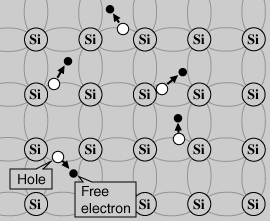# 1. Intrinsic Carrier Concentration

In an undoped semiconductor, or in a semiconductor without impurities, there must be charge neutrality. Therefore, an ideal semiconductor has equal numbers of holes and conductive electrons, because they must obey the equationandwhere p is the number of free holes and n is the number of free electrons in the material. Because the number of holes is equal to the number of electrons, the concentration of each is equal to some quantity ni. We’ll call this quantity the intrinsic carrier concentration, and the pure semiconductor material we’ll call intrinsic material. This gives us a basis for understanding the doped case, which we’ll come to in the next section.

Keep in mind that a distinct feature of semiconductors is that it only takes a little thermal excitation (higher temperature) to excite some electrons from the valence to the conduction band. Therefore, it becomes more and more conductive at higher temperatures. Therefore, the intrinsic carrier concentration of a semiconductor varies with temperature – higher temperature, more “freed” electrons and more holes (vacancies). To clarify, when we mean charge carriers, we are only referring to free electrons.

Furthermore, the intrinsic carrier concentration also depends on the band gap, because this determines how easily electrons become conductive. Thus, we have that the intrinsic carrier concentration is already tied to solar cell efficiency, because in order to maximize conductivity we want to consider both the band gap and temperature of the material at which the most electrons are freed for conductivity. We will see later how the “freeing” of electrons also comes about through sunlight absorption.